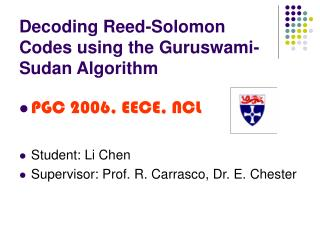# Decoding Reed-Solomon Codes using the Guruswami-Sudan Algorithm - PowerPoint PPT PresentationDownload PresentationDecoding Reed-Solomon Codes using the Guruswami-Sudan Algorithm

Decoding Reed-Solomon Codes using the Guruswami-Sudan AlgorithmDownload Presentation## Decoding Reed-Solomon Codes using the Guruswami-Sudan Algorithm

- - - - - - - - - - - - - - - - - - - - - - - - - - - E N D - - - - - - - - - - - - - - - - - - - - - - - - - - -
##### Presentation Transcript

1. Decoding Reed-Solomon Codes using the Guruswami-Sudan Algorithm • PGC 2006, EECE, NCL • Student: Li Chen • Supervisor: Prof. R. Carrasco, Dr. E. Chester

2. Introduction • List Decoding • Guruswami-Sudan Algorithm • Interpolation (Kotter’s Algorithm) • Factorisation (Ruth-Ruckenstein Algorithm) • Simulation Performance • Complexity Analysis • Algebraic-Geometric Extension • Conclusion

3. Funny Talk about List Decoder • Decoder—Search the lost boy named “John” • Unique decoder—Police without cooperation • List decoder—Police with cooperation Police Decoder from now

4. List Decoding • Introduced by P. Elias and J. Wozencraft independently in 1950s • Idea: • Unique decoder can correct r1, but not r2 • List decoder can correct r1 and r2

5. Reed-Solomon Codes • Encoding: k n(k<n) (C0, C1, …, Cn-1)=(f(x0), f(x1), …, f(xn-1)) transmitted message f(x)=f0x0+f1x1+∙∙∙+fk-1xk-1 kdimensional monomial basis of curve y=0 • Application: Storage device Mobile communications

6. 1999 1997 Guruswami-Sudan Algorithm

7. GS Overview • Decode RS(5, 2): • Encoding elemnts x=(x0, x1, x2, x3, x4) • Received word y=(y0, y1, y2, y3, y4) Build Q(x, y) that goes through 5 points: Q(x, y)=y2-x2y-(-x) y-p(x)?=f(x) y-x Q(x, y) has a zero of multiplicity m=1 over the 5 points. GS = Interpolation + Factorisation The Decoded codeword is produced by re-evaluate p(x) over x0, x1, x2, x3, x4!!!

8. How about increase the degree of Q(x, y)? • Q2=(y2-x2)2y-(-x) y-x y-p(x)?=f(x) y-(-x) y-x Q2(x, y) has a zero of multiplicity m=2 over the 5 points. The higher degree of Q(x, y) more candidate to be chosen as f(x) diverser point can be included in Q(x, y) better error correction capability!!!

9. GS Decoding Property • Error correction upper bound: (1) Multiplicity m Error correction tm Output list lm • Examples: • RS(63, 15) with r=0.24, e=24 RS(63, 31) with r=0.49, e=16

10. Interpolation---Build Q(x, y) • Multiplicity definition: (2) ---qab=0 for a+b<m, Q has a zero of multiplicity m at (0, 0). • Define over a certain point (xi, yi): • ---quv=0 for u+v<m, Q has a zero of multiplicity m at (xi,yi) • quv is the Q’s (u, v) Hasse derivative evaluation on (xi, yi) • (3)

11. Cont… • Therefore, we have to construct a Q(x, y) that satisfies: Q(x, y)=min{Q(x, y)Fq[x, y]|DuvQ(xi, yi)=0 for i=0, ∙∙∙, n-1 and u+v<m} Q has a zero of multiplicity m over the n points

12. Kotter’s Algorithm • Initialisation: G0={g0, g1, …, gj, …,} Hasse Derivative Evaluation If i=n, end! Else, update i, and (u, v) Find the minimal polynomial in J: Bilinear Hasse Derivative modification: For (jJ), if j=j*, if j≠j*,

13. Factorisation---Find p(x) • p(x) satisfy: y-p(x)|Q(x, y) and deg(p(x))<k • p(x)=p0+p1x+∙∙∙+pk-1xk-1 ---we can deduce coefficients p0, p1, …, pk-1sequentially!!!

14. Ruth-Ruckenstein Algorithm Q0(x, y) Q1(x, y) Q2(x, y) p(x) p(x) Q’s sequential transformation: (4) pi are the roots of Qi(0, y)=0.

15. Simulation Results 1----RS(63, 15) AWGN Rayleigh fading Coding gain: 0.4-1.3dB 1-2.8dB

16. Simulation Result 2----RS(63, 31) AWGN Rayleigh fading Coding gain: 0.2-0.8dB 0.5-1.4dB

17. Complexity Analysis RS(63, 15) RS(63, 31) Reason: Iterative Interpolation

18. Little Supplements----GS’s AG extension RS: f(x)Q(x, y)p(x) AG: f(x, y)Q(x, y, z) p(x, y)

19. Conclusion of GS algorithm • Correct errors beyond the (d-1)/2 boundary; • Outperform the unique decoding algorithm; • Greater potential for low rate codes; • Used for decode AG codes; • Higher decoding complexity----Need to be addressed in future!!!